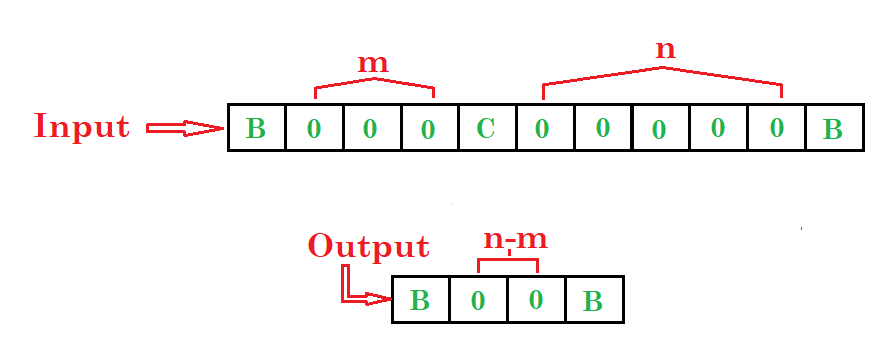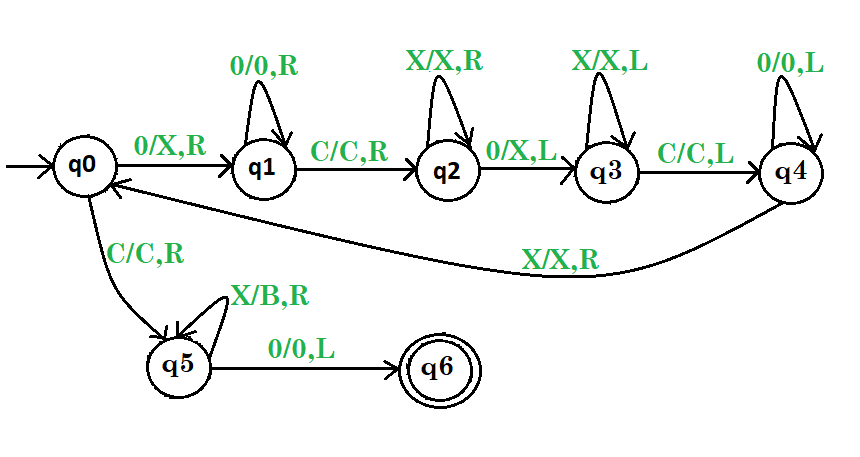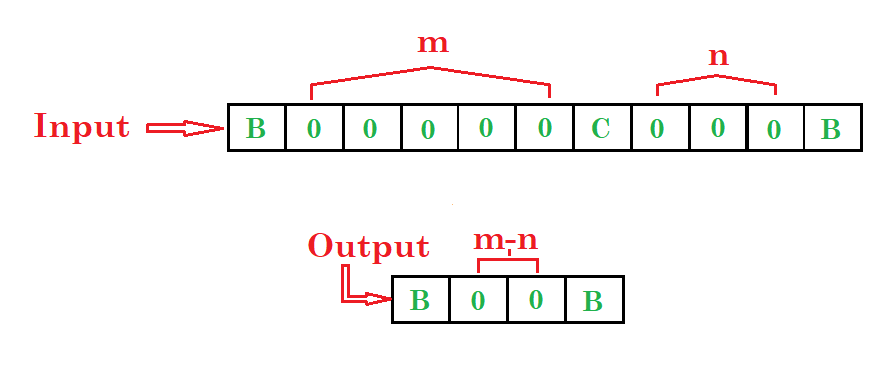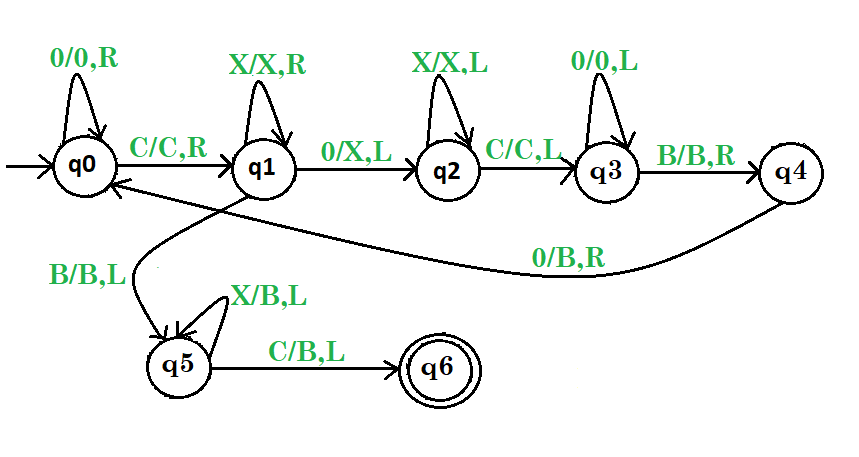# Turing machine for subtraction | Set 1

Prerequisite – Turing Machine
Problem-1: Draw a Turing machine which subtract two numbers.

Example:Steps:

• Step-1. If 0 found convert 0 into X and go right then convert all 0’s into 0’s and go right.
• Step-2. Then convert C into C and go right then convert all X into X and go right.
• Step-3. Then convert 0 into X and go left then convert all X into X and go left.
• Step-4. Then convert C into C and go left then convert all 0’s into 0’s and go left then convert all X into X and go right and repeat the whole process.
• Step-5. Otherwise if C found convert C into C and go right then convert all X into B and go right then convert 0 into 0 and go left and then stop the machine.Here, q0 shows the initial state and q1, q2, q3, q4, q5are the transition states and q6shows the final state.
And X, 0, C are the variables used for subtraction and R, L shows right and left.

Problem-2: Draw a Turing machine which subtract two numbers m and n, where m is greater then n.Steps:

• Step-1. If 0 found convert all 0’s into 0’s and go right then convert C into C and go right
• Step-2. If X found then convert all X into X and go right or if 0 found then convert 0 into X and go left and go to next step otherwise go to 5th step
• Step-3. Then convert all X into X and go left then convert C into C and go left
• Step-4. Then convert all 0’s into 0’s and go left then convert B into B and go right then convert 0 into B and go right and repeat the whole process
• Step-5. Otherwise if B found convert B into B and go left then convert all X into B and go left then convert C into B and go left and then stop the machine.Here, q0 shows the initial state and q1, q2, q3, q4, q5are the transition states and q6shows the final state.
And B, X, 0, C are the variables used for subtraction(m>n) and R, L shows right and left and B variable is a input symbol.

Don’t stop now and take your learning to the next level. Learn all the important concepts of Data Structures and Algorithms with the help of the most trusted course: DSA Self Paced. Become industry ready at a student-friendly price.

My Personal Notes arrow_drop_upCheck out this Author's contributed articles.

If you like GeeksforGeeks and would like to contribute, you can also write an article using contribute.geeksforgeeks.org or mail your article to contribute@geeksforgeeks.org. See your article appearing on the GeeksforGeeks main page and help other Geeks.

Please Improve this article if you find anything incorrect by clicking on the "Improve Article" button below.